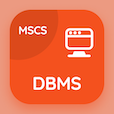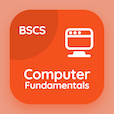Computer Science Courses Online

Digital Logic Design Certification Exam Tests

Digital Logic Design Practice Test 31

# Introduction to Combinational Logics Quiz Answers PDF - 31

The Book Introduction to Combinational Logics Quiz Questions and Answers, introduction to combinational logics Quiz MCQs PDF, chapter 6-31 to download online logic design degree courses. Study Combinational Logics MCQ Questions PDF, introduction to combinational logics Multiple Choice Questions (MCQ Quiz) for online college degrees. The e-Book Introduction to Combinational Logics Quiz App Download: introduction to combinational logics, five variable map, memory units, registers in dld, control implementation in asm test prep for top computer science schools in the world.

The Quiz: Logic gates takes input signals and generates signals to PDF, "Introduction to Combinational Logics" App (Android & iOS) Free with input, within gate, output, and circuit choices for 2 year computer science degree. Practice combinational logics questions and answers, Google eBook to download free sample for CS major.

## Logic Design Quiz Online: Introduction to Combinational Logics MCQs - 31

MCQ: Logic gates takes input signals and generates signals to

A) within gate
B) input
C) output
D) circuit

MCQ: A six Variable map needs

A) 32 Squares
B) 40 Squares
C) 50 Squares
D) 64 Squares

MCQ: For writing operation on the memory

A) ME=0
B) WE=0
C) ME=1
D) both a and b

MCQ: Transformation of information into registers is called

B) gated latch
C) latch
D) storing

MCQ: One that is not present in the list of the state table is

A) present state
B) input
C) next state
D) previous state

### Mock Tests: Digital Logic Design Course Prep

Download Digital Logic Design Quiz App, DataBase Management System (MCS) MCQ App, and Computer Fundamentals MCQs App to install for Android & iOS devices. These Apps include complete analytics of real time attempts with interactive assessments. Download Play Store & App Store Apps & Enjoy 100% functionality with subscriptions!